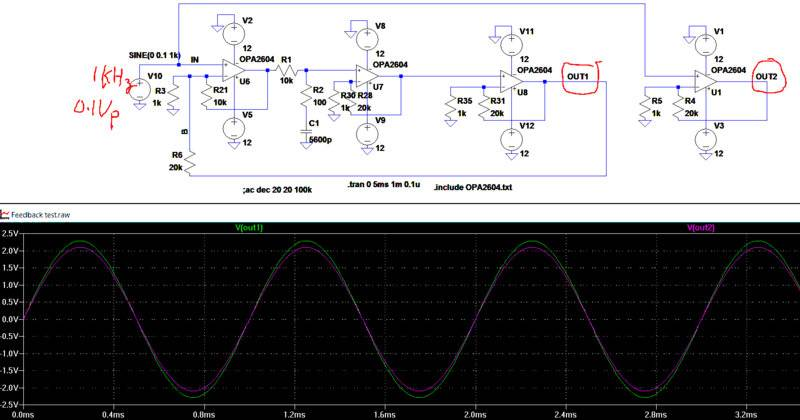# How do you analyze this circuit

This in not homework. I am playing with this circuit and I don't understand what is not right. Here are the circuits.a) I "built" an opamp out of U6, U7 and U8. I set the gain to 21 by R6/R3+1=20+1=21. The output is OUT1. But I get a little more gain than expected, like 22 or 23.

b) I use U1 as reference as set gain = R4/R5 + 1 = 21, the output is OUT2.

The signal generator is V10, frequency is 1KHz, 0.1Vpeak. You can see the graph OUT2 ( red) is correct at 21, and OUT1(green).

Am I thinking wrong that this is not the right way to do feedback?

Thanks

Alan

#### Attachments

Last edited:

Homework Helper
Gold Member
2020 Award
Getting a gain of 22 or 23 vs. the expected 21 could simply be the result of resistor tolerances. Unless you use precision resistors, they are not very precise. ## \\ ## @jim hardy Might you have an input on this one?

Getting a gain of 22 or 23 vs. the expected 21 could simply be the result of resistor tolerances. Unless you use precision resistors, they are not very precise. ## \\ ## @jim hardy Might you have an input on this one?
This is a simulation using LTSpice. It is quite consistent.

Basically I am designing a hifi amp with tube front end section. Due to the inverted nature of the common cathode of tubes, I have to have either two or 4 stages. Two stages don't have enough gain(loopgain of only 56dB), 4 stages have too much gain. It's hard to have 3 stages because of the inverting nature that will make the input impedance too low ( like inverted opamp that input impedance is the gain resistor). So I want to have a non inverting opamp as a front end stage to get some gain ( say loop gain of about 80dB at low frequency).

The problem is in order to have NFB, I need the -ve input to feed back to. This is what I am trying to do, is to have R6 as feedback resistor, R3 is the gain setting resistor to give gain = R6/R3+1=21. But if you look at the circuit, it does look a little funny as U6 has both local feedback(from R21) and global feedback from R6.

I am just guess in this design. I am not 100% sure this is even correct. That's why I come here to let people look at this. If someone has an idea how to close the loop to have negative feedback, I am all ears ( eyes).

I can use transistor differential pair as front end that will give me both +ve and -ve input. But that's a lot more circuit for my taste.

Again, this is not a homework. This is real life design that I eventually will build a complete amp. If anyone use LTSpice IV or XVII, I can try to upload the .asc file.
Thanks

Alan

Last edited:
Tom.G
I get a gain of approx. 22.999.
You used R6/R3+1=21.
It should be R6/(R3||R21)+1 =20/.90909+1 =23

Cheers,
Tom

•essenmein, jim hardy and yungman
I get a gain of approx. 22.999.
You used R6/R3+1=21.
It should be R6/(R3||R21)+1 =20/.90909+1 =23

Cheers,
Tom

I think you are right, When I reduce R21 to 500ohm, R21//3 =333ohm and theoretical gain is 61. I got about 56 in the simulation mainly because of low loop gain that cause error. I tried R21= 1K, I got gain about 40. The theoretical gain is 41.

Looks like my idea works, just calculating the gain wrong. I did not think of the output of U6 should be considered as ground.

Thanks

EDIT: if this is the way I look at this, then the internal gain of U6 should be R21/(R3//R6). that is in the schematic gain of U6 is 10K/(1K//20K)+1= 10K/0.9524K+1=11.5. So I add about 21.2dB of loop gain. 56+21=77dB of loop gain is respectable. THD should be low enough.

That's great.

Alan

Last edited:
berkeman
Mentor
this is not the right way to do feedback?
You need to be careful connecting feedback across multiple stages like that. The parasitic capacitances and delay through each opamp in that circuit will introduce additional phase shifts, and likely erode your phase margin to the point where you can get oscillations. You should include some typical parasitic capacitance at each node and check how your phase margin changes, IMO...

•Averagesupernova